## Example Questions

### Example Question #781 : Geometry

Find the diameter of a sphere with a surface area of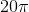.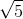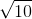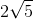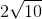Explanation:

Write the formula to find the surface area of a sphere.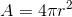Substitute the area and solve for the radius.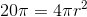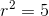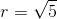The diameter is double the radius.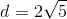### Example Question #2 : Spheres

What is the diameter of a sphere if the surface area is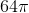?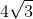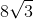Explanation:

Write the formula for the surface area of a sphere.Substitute the area and find the radius.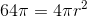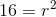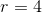The diameter is double the radius.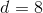### Example Question #3 : Spheres

What is the diameter of a sphere with a volume of?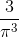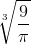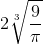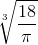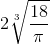Explanation:

Write the formula for the volume of a sphere.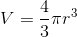Substitute the volume.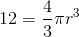Multiply by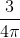on both sides in order to isolate the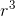term.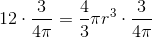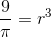Cube root both sides.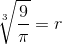The diameter is double the radius.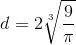The answer is: